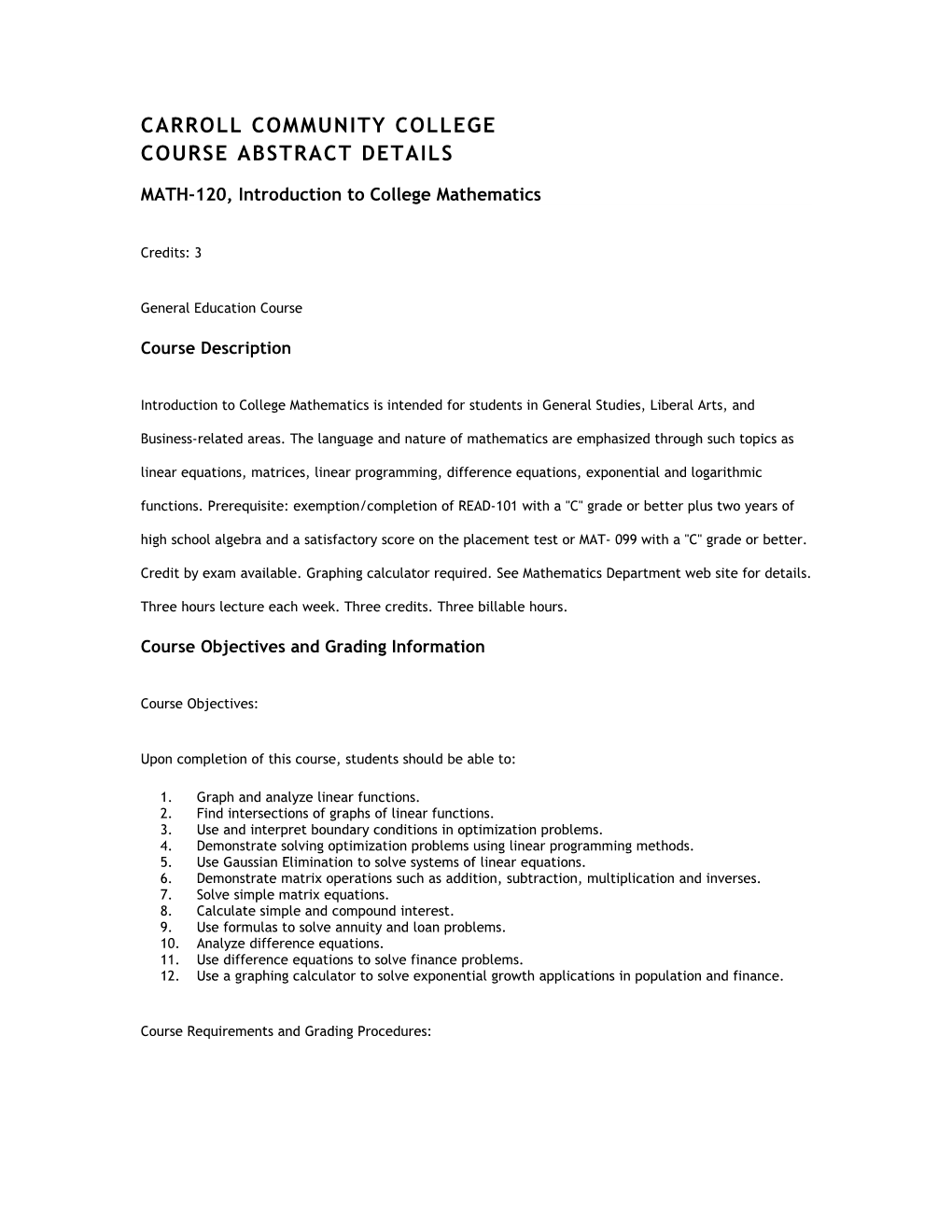# Carroll Community College### Course Abstract Details

#### MATH-120, Introduction to College Mathematics

Credits: 3

General Education Course

###### Course Description

Introduction to College Mathematics is intended for students in General Studies, Liberal Arts, and Business-related areas. The language and nature of mathematics are emphasized through such topics as linear equations, matrices, linear programming, difference equations, exponential and logarithmic functions. Prerequisite: exemption/completion of READ-101 with a "C" grade or better plus two years of high school algebra and a satisfactory score on the placement test or MAT- 099 with a "C" grade or better. Credit by exam available. Graphing calculator required. See Mathematics Department web site for details. Three hours lecture each week. Three credits. Three billable hours.

###### Course Objectives and Grading Information

Course Objectives:

Upon completion of this course, students should be able to:

1. Graph and analyze linear functions.
2. Find intersections of graphs of linear functions.
3. Use and interpret boundary conditions in optimization problems.
4. Demonstrate solving optimization problems using linear programming methods.
5. Use Gaussian Elimination to solve systems of linear equations.
6. Demonstrate matrix operations such as addition, subtraction, multiplication and inverses.
7. Solve simple matrix equations.
8. Calculate simple and compound interest.
9. Use formulas to solve annuity and loan problems.
10. Analyze difference equations.
11. Use difference equations to solve finance problems.
12. Use a graphing calculator to solve exponential growth applications in population and finance.